ENGR338 Lab 2022 Fall

Introduction: This lab uses cadence tools and software to build and layout simple gates.

Methods: First cadence was setup by following the initial tutorial, I didn't take any photos of this because it didn't feel like much of an accomplishment, more of a stepping stone to the real lab.

The first step was creating a simple voltage divider circuit using 10k ohm resistors. This can be seen below in Figure 1.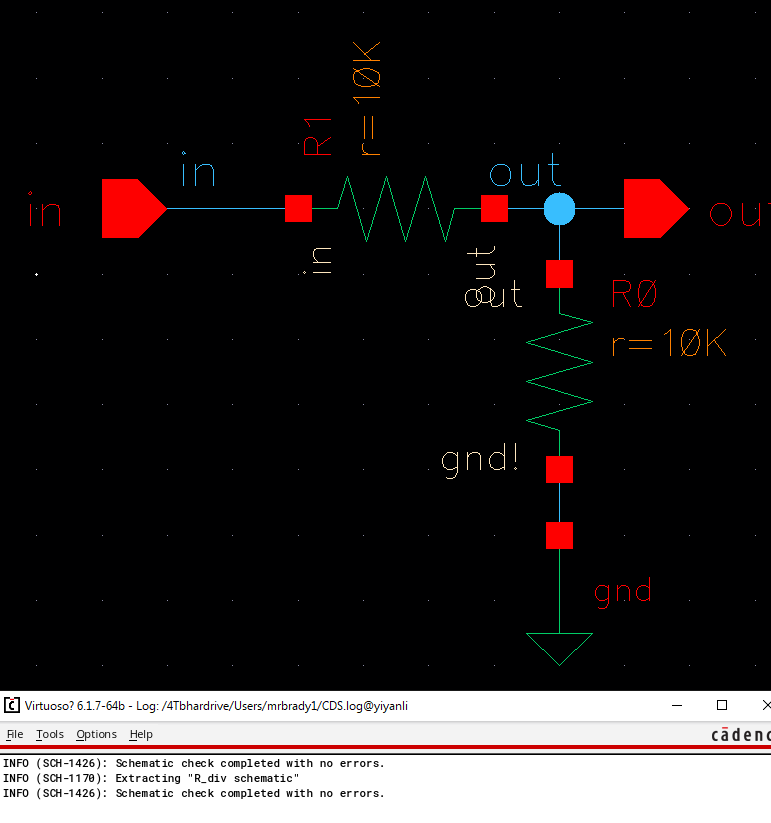Figure 1. Voltage Divider Schematic.

Pins were then added to the schematic on the in and out pins as seen below in Figure 2.Figure 2. Schematic with import/export pins

A symbol view was then created for the design and added into another schematic which included a new dc voltage source and a few auxillary wires.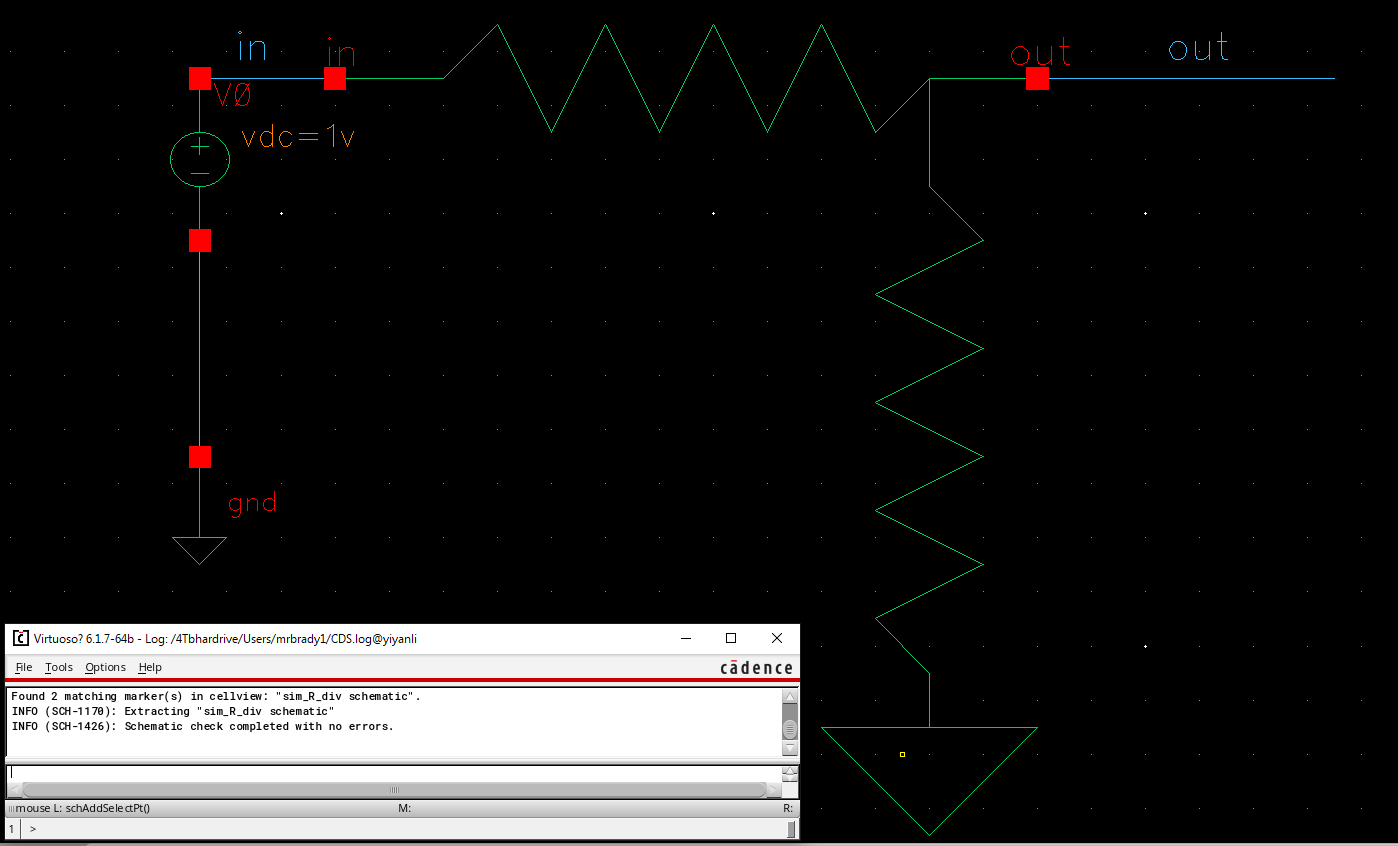Figure 3. Schematic using voltage divider icon.
The voltage divider layout was then created by first placing an n-well and stretching it to 56um by 4.5um. Then two ntaps were added to the ends of the resistor so that arcs could be connected and res_id was dragged to cover the resistor. This resistor was duplicated and used arcs to connect the two terminals creating the vout network and arcs on the left terminals creating the vdd and ground connections. The layout can be seen below in Figure 4.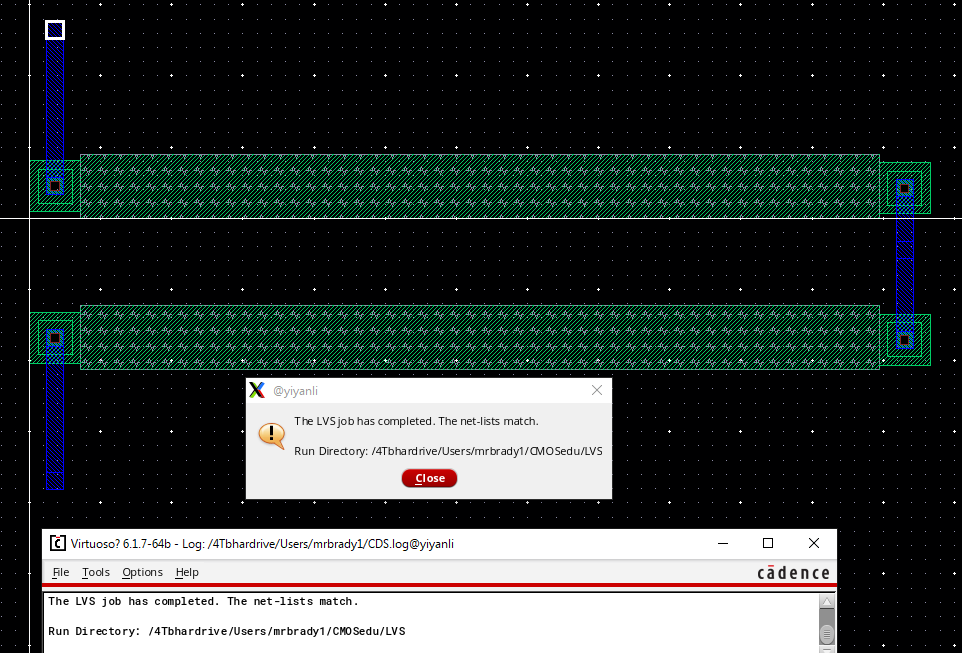Figure 4. Voltage divider layout with LVS check.

Now for the real deal. An NMOS was placed in the schematic area with in/out pins connecting to the GDS. The width was set to 6um and the length to 600n. The schematic can be seen below in Figure 5.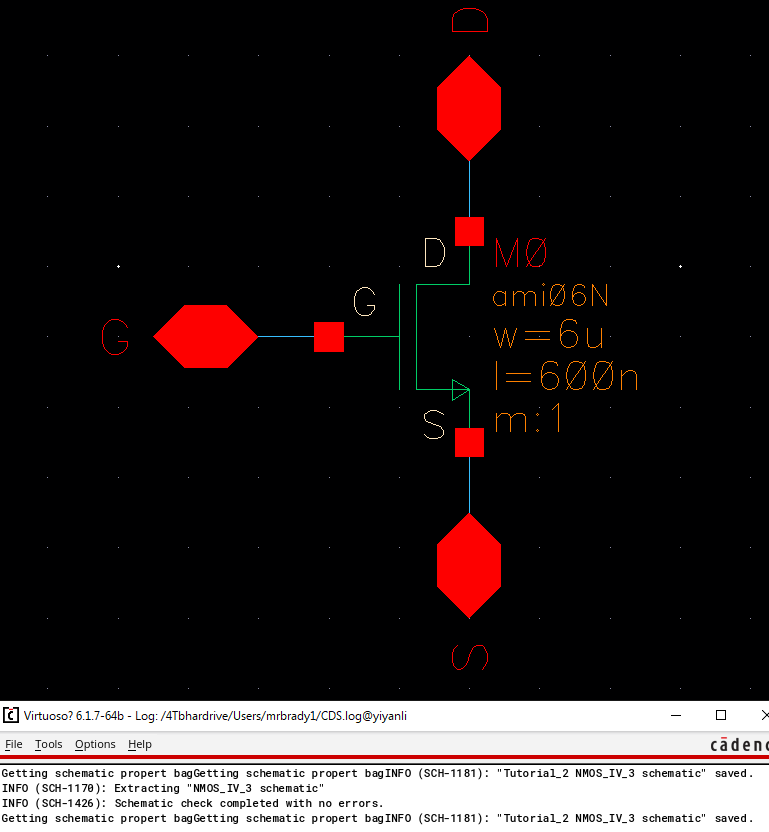Figure 5. Nmos with pins schematic.

A new icon was then created for the new 6u/600n NMOS as seen below in Figure 6.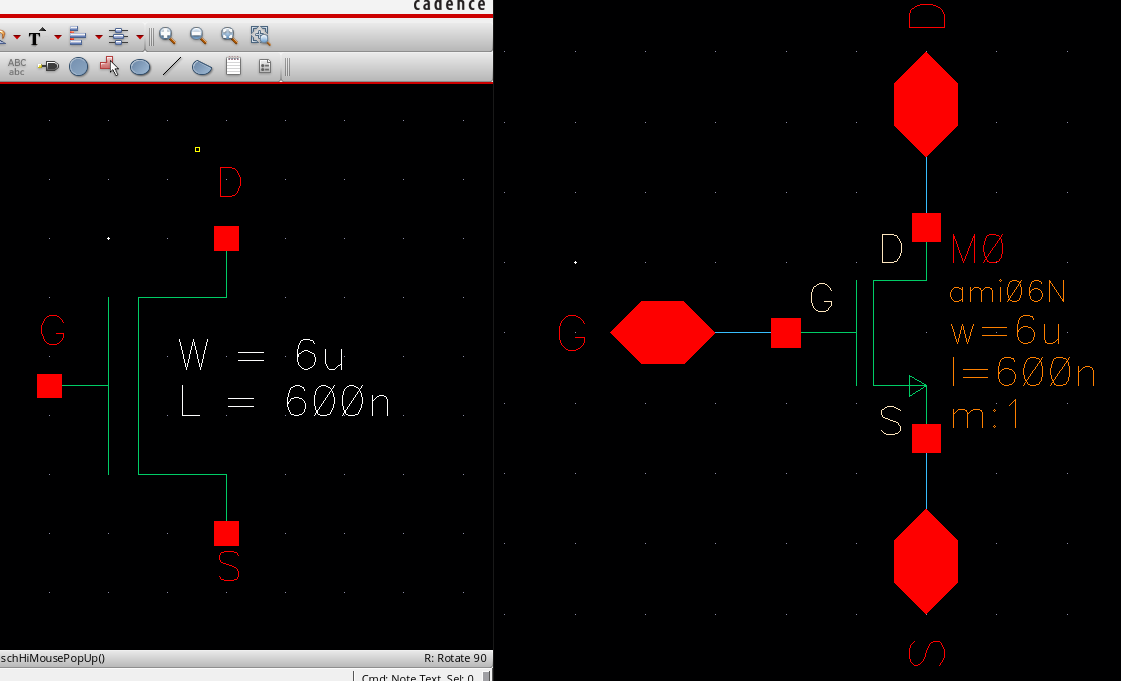Figure 6. Icon view(left) of 6um by 600n NMOS gate(right).

Then everybody's favourite part, the layout was created. This was fairly straight forward except I was having trouble connecting my arcs to the NMOS drain and sink, they kept not wanting to make an actual connection and then had overlap errors. After deleting and replacing the arc several times however it seemed to eventually work. A ptap and a poly-metal1 via was placed. The gate was wired to the via and the sink/drain were wired to pins labeled accordingly. The via was also given a pain appropriately labeled G. The ptap was labeled ground.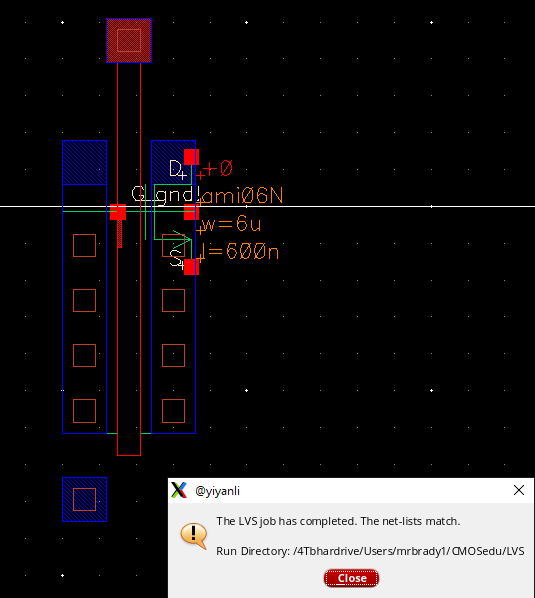Figure 7. NMOS gate passing LVS.

The same process was repeated for PMOS and can be seen below. The Icon and Schematic were created for the PMOS in an identical way using the existing PMOS gate with a width of 12u and 600n for length. 2-way pins were shorted to each terminal and labeled accordingly. The result can be seen below in Figure 8.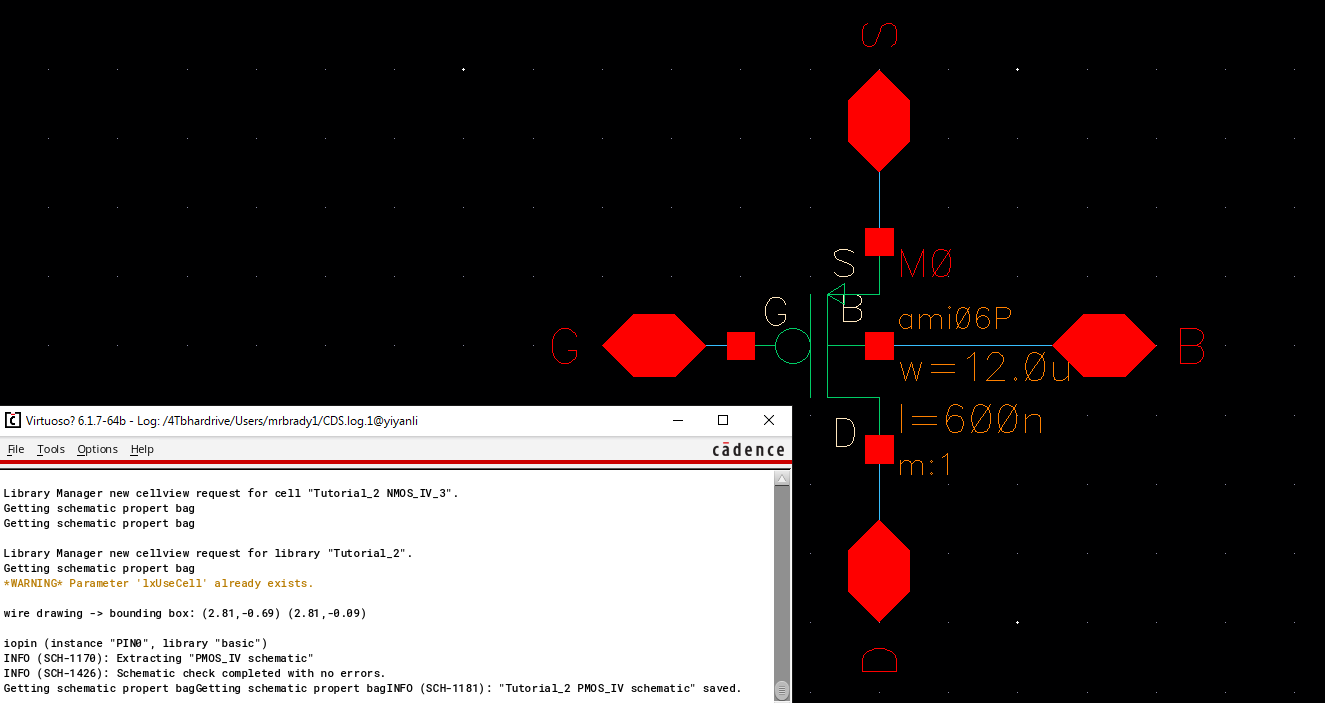Figure 8. PMOS Schematic.

The icon was also almost identical except with a small circle ontop. It can be seen below in Figure 9.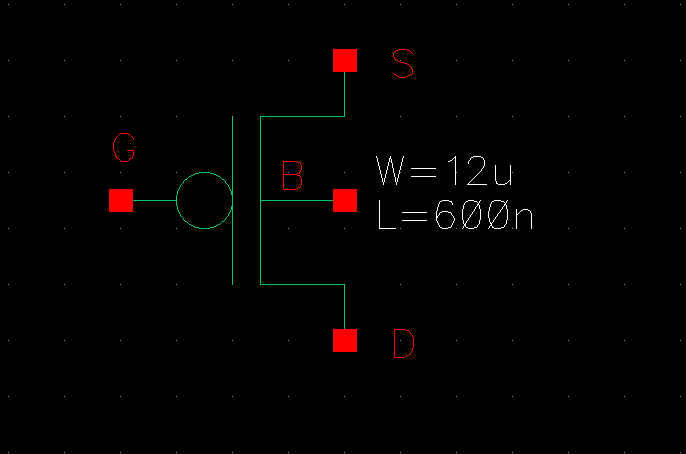Figure 9. PMOS icon.

Finally, it was laid out using an ntap instead of ptap and everything else being the same.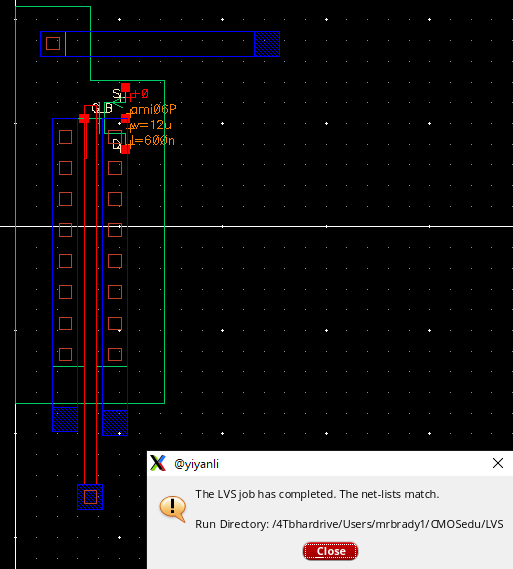Figure 10. PMOS layout.

Results:

The voltage divider, NMOS, and PMOS were all simulated and the results were as expected. For each simulation the extracted layout view was also simulated and yielded identical results so I didn't take an extra screenshot.

The voltage divider was simulated using a spectre simulation and a constant vdd of 1V. The simulation can be seen below in Figure 11.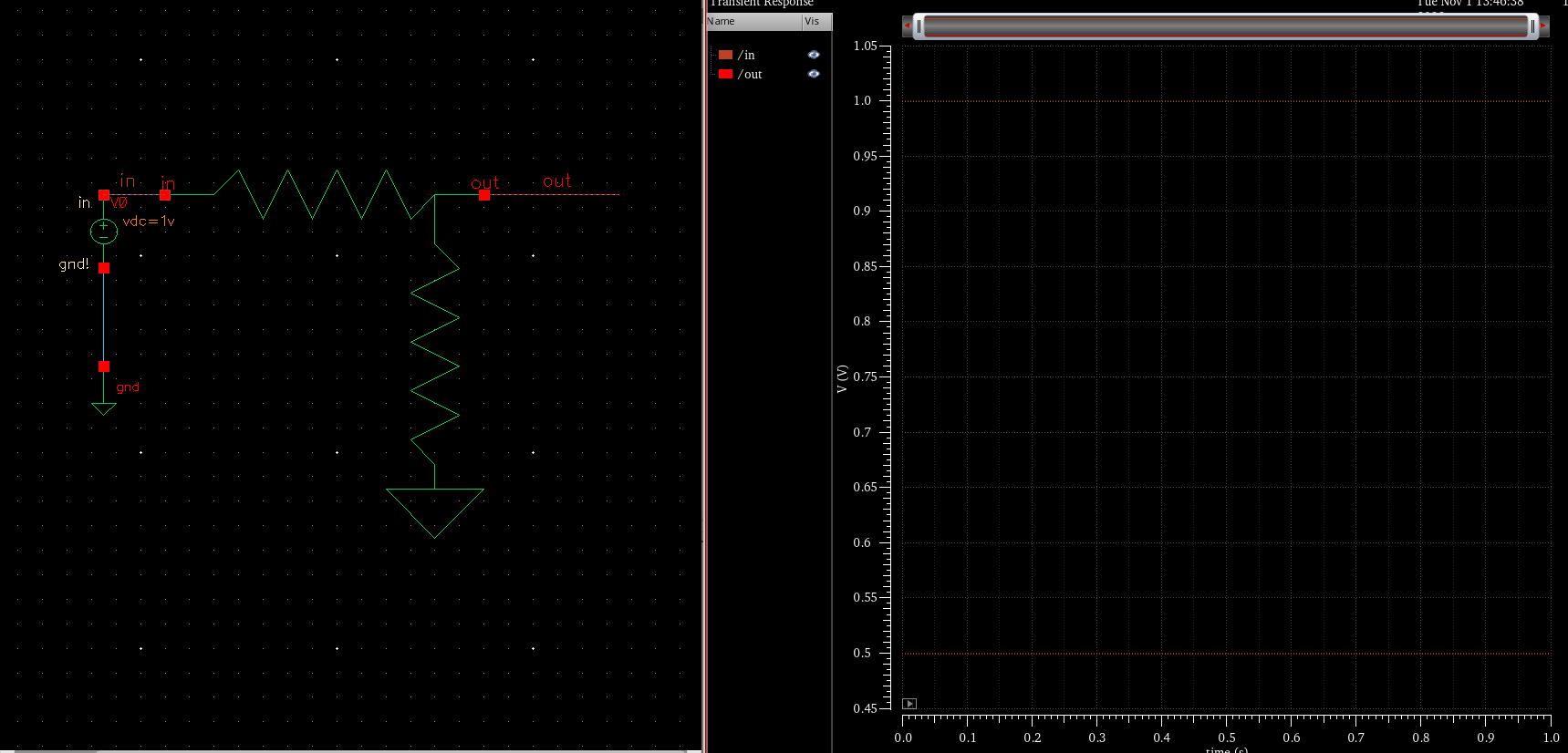Figure 11. Voltage divider simulation input 1V and 0.5V output.

A parametric linear sweep Spectre analysis was used for the PMOS and NMOS to create IV curves with varrying gate voltages. The NMOS curves can be seen below in Figure 12. where the gate voltage ranged from 0 to 5V.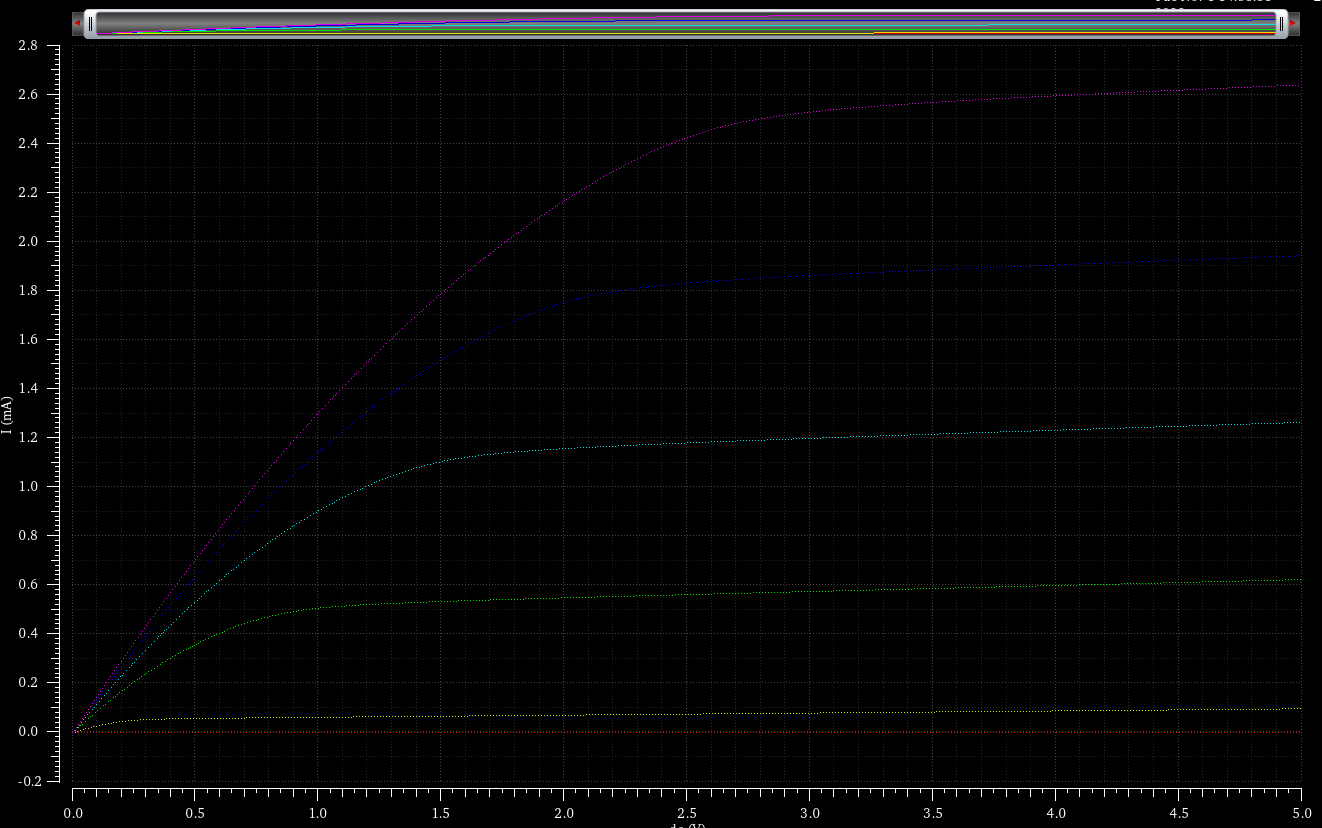Figure 12. NMOS simulation

The same process was used to simulate the PMOS.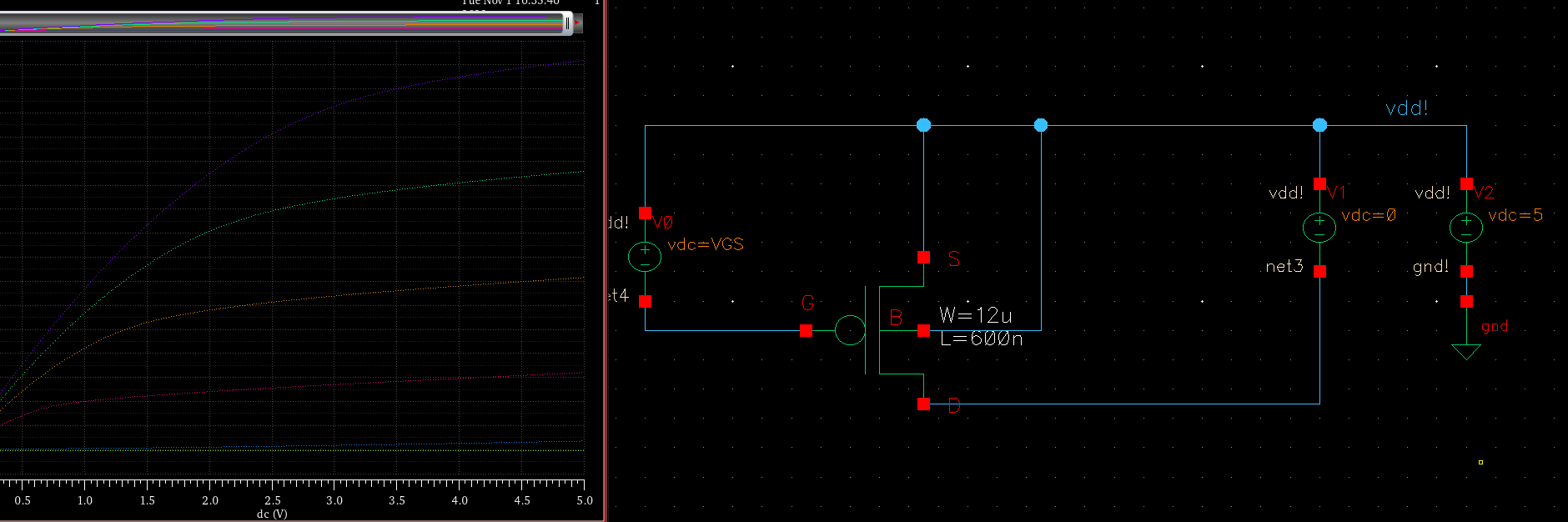Figure 13. PMOS simulation results

Discussion: It was cool to use the industry standard software but it was tricky to learn all the nuances especially when it came to creating simulations.